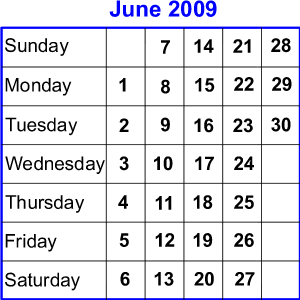You may also likePrompt Cards

These two group activities use mathematical reasoning - one is numerical, one geometric.Consecutive Numbers

An investigation involving adding and subtracting sets of consecutive numbers. Lots to find out, lots to explore.Exploring Wild & Wonderful Number Patterns

EWWNP means Exploring Wild and Wonderful Number Patterns Created by Yourself! Investigate what happens if we create number patterns using some simple rules.

Today's Date - 01/06/2009

Today's Date - 01/06/2009This problem will be published for the first time on 1 June 2009, not an exciting date perhaps (even when written as $01$.$06$.$09$).

But in two days' time it will be $03.06.09$! Why do you think I might find that more exciting?

Earlier this year we had $8$ January $2009$ or $08$.$01$.$09$ ($8 + 1 = 9$).

There'll be some more like that this year. Can you find them?

Consider the following: $29$ March $1987$, or if you prefer $29$.$03$.$87$, or $3$.$29$.$87$
and then
$8$ November $1988$ or $8$.$11$.$88$

(Maybe one of these was the day someone you know was born.)

This time, $29 \times 3 = 87$ and $8 \times 11 = 88$.

We could also look at $27$ March $2009$ or $27$.$03$.$09$ as $27 \div 3 = 9$.
And we had the $18$ February or $18$.$02$.$09$ and that could be $18 \div 2 = 9$

All these may be called special 'productive' dates because we can find a mathematical relationship between the day, month and year.

I wonder which year, irrespective of the century, has the most 'productive' dates?

You could find all the calculations that work for the current year (if you are looking at this problem in years to come, it won't be 2009, but that doesn't matter!).

Why do this problem?

This activity is a good number exploration. It gives pupils the opportunity to show the number relationships that they are able to use and therefore is a 'low threshold high ceiling' task. It also allows pupils to develop their own methods of approach for such an open activity. It could lead to conversations about estimating and working systematically as pupils explain their ways of tackling the challenge.

Possible approach

Sometimes it's good to start an activity apparently out of "thin air". So you might choose a pupil's birthday that has some potential, or save the activity until the date has some possibilities. Write the date on the blackboard - to celebrate the pupil's birthday or because it's that day's date - and then say "Oh look I think there may be something special about that date!". This will initiate excitement and discussion.

You can lead into the activity as shown on this site at whatever level suits the group best. Younger or less experienced learners may be happy to begin with addition and subtraction, but the beauty of the activity is that children can show you what they know as they are not constrained.

This challenge would lend itself to being a 'simmering activity' so that you could have a space on the wall where children can post up further findings over a week or more.

Key questions

How have you decided where to look for dates that will be interesting?

Possible extension

What about taking in the time as well?
At $1$:$41$ on $7/7/2009$ could be $1 \times 41 \times 7 \times 7 = 2009$
so, $7$:$41$ on $1/7/2009$ would be $7 \times 41 \times 7 \times 1 = 2009$
(Years with many solutions are $2016, 2040, 2052, 2070, 2080$ and $2088$ and some may like to follow up the fact that these years are also just 1 away from a prime!)
Alternatively, learners might like to try the How Many Times? problem which investigates consecutive numbers appearing on a digital clock.

Possible support

Some pupils will find it necessary to have cards with numerals on to move about in front of them and some will feel more confident if they have the use of a calculator.